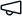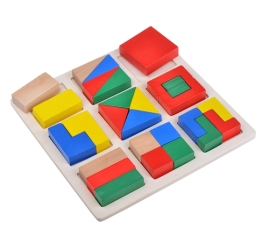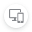Give us feedback# Logic Exercises

Stable version 1.0.0 (Compatible with OutSystems 11)
5.0
(1 rating)# Logic Exercises

Details
Simple Assignments of finding pallindrome, fibonacci and armstrong number

In this component we are having exercises of finding

1. finding a given number is pallindrome or not.

2. finding a given number is armstrong number or not

3. finding nth fibonacci number of original fibonacci series

4. finding n-series fibonacci numbers from the original sequence

5. using of an api and getting data from api (ex: catwiki api with sample screens and data)

Release notes (1.0.0)